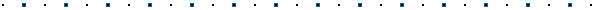#Written by Robert Dunlop Microsoft DirectX MVPTarget Version:

 Related Articles of Interest: Using W-Buffers

### Introduction

Anyone who has rendered a large scene has likely had to fight issues of depth buffer resolution, and the effects of the non-linearity of Z-buffering (for a bit of numeric background, see Using W-Buffers).  The resulting depth artifacts often must be dealt with by limiting the depth range of the viewing frustum, a solution that is not always ideal especially in large outdoor scenes.  The use of W-buffering offered some promise, with better distribution of depth values, but hardware support has been limited and does not look to be supported in future hardware.

In this article we'll look at an easy way to implement linear depth values using a Z-buffer, by implementing transformation in a programmable vertex shader.  Benefits and features of this method include:Linear distribution of depth values, resulting in reduced depth artifacts of distant objects.Method may be modified to generate custom distribution curves, for example to provide some additional resolution at near distances without the major non-linearity of Z-buffers (not covered in this article).Requires only 1-2 additional vertex shader instructions compared to conventional transformation.Allows for greater far plane distances than normal non-linear Z-buffer distribution.

### Projection Transform, Perspective Division, and Non-Linearity

To begin with, let's take a look at the transformation process, and how depth values are manipulated to get the final value that gets written to our depth buffer.  If we are using the fixed function pipeline, we can consider the process in three parts:

Setup of the Projection Matrix

A perspective projection matrix is usually set up in the form:
 w 0 0 0 0 h 0 0 0 0 Q 1 0 0 -QN 0
Where:

w = X scaling factor
h = Y scaling factor
N = near Z
F = far Z
Q = F / (F-N)

Transformation of 3D vertex coordinates to 4D homogeneous coordinates

While vertices are transformed by the combined world, view, and projection matrices, we are going to focus here solely on the effect of the projection matrix.  Given vertex coordinates v(x,y,z,1) that have been transformed to camera space, multiplying by the projection matrix will result in a 4D vertex:

V' = v * projectionMatrix

There are two functions of this transformation that are important to note:If you simplify the V'.z result, you will find that the configuration of the projection matrix results in a linear function such that f(N) = 0 at the near plane, and f(F) = F at the far plane.V'.w = v.z, i.e. the camera space Z value is preserved in the fourth component of the result.

At this point, all components still have a linear relationship with camera space.

Projection to 4D non-homogeneous coordinates: division by W'

Following transformation, the X, Y, and Z coordinates are divided by W, and 1/W (reciprocal of homogenous W, aka RHW) is stored in the fourth component of the transformed vertex position:

Vout (X,Y,Z,RHW) = (V'.x/V'.w, V'.y/V'.w, V'.z/V'.w, 1/V'.w)

Since the previous step (transformation by the projection matrix) resulted in a Z that ranges from 0 -> Far over the range of Near -> Far, the resulting Z value is scaled to a range of 0.0 -> 1.0:

 Camera Z V'.z V'.w Vout.z Near 0.0 Near 0.0 / Near = 0.0 Far Far Far Far / Far = 1.0

Unfortunately, it is this final division that causes the non-linearity of transformed depth values.  For example, given a near plane of 10.0 and a far plane of 10000.0:

 Camera Z V'.z V'.w Vout.z 10.0 0.0 10.0 0.0 100.0 90.09009 100.0 0.900901 500.0 490.4905 500.0 0.980981 1000.0 990.991 1000.0 0.990991 10000.0 10000.0 10000.0 1.0

### Customizing the Projection in a Vertex Shader

When using a programmable vertex shader, we have direct control of the transformation process, and can implement our own.  Vertex position can be read from the input registers, manipulated however we like, then output as a 4D homogenous coordinate to the output position register.  However, there is one apparent problem at handling our linearity issue: the output from the shader is still homogenous, and will be divided in the same manner as the output from the fixed pipeline transformation would be.  So how do we handle this, if we can't eliminate the division operation?

The answer is actually pretty simple - just multiply Z by W prior to returning the result from the vertex shader.  The net effect is that Z*W/W = Z!  If we first divide Z by the far distance, to scale it to the range of 0.0 -> 1.0, we've got a linear result that will survive perspective division.  A simple HLSL implementation might look (in part) something like this:

```float4 vPos = mul(Input.Pos,worldViewProj);
vPos.z = vPos.z * vPos.w / Far;
Output.Pos = vPos;```

To simplify this, instead of needing to divide by the far plane distance to scale Z, we could instead scale the values in the Z column of the projection matrix we use:

```D3DXMATRIX mProj;
D3DXMatrixPerspectiveFovLH(&mProj,fFov,fNear,fFar);
mProj._33/=fFar;
mProj._43/=fFar;
//...set to shader constant register or concatenate
//...with world and view matrices first as needed```

This reduces the vertex shader transformation to:

float4 vPos = mul(Input.Pos,worldViewProj);
vPos.z = vPos.z * vPos.w;
Output.Pos = vPos;

### The results...

Going back to our previous scenario (near = 10.0, far = 10000.0), here are the resulting depth values that would be generated, assuming that the projection matrix were scaled as noted previously:

 Camera Z V'.z V'.w V'.z * V'.w Vout.z 10.0 0.0 10.0 0.0 0.0 100.0 0.009009 100.0 0.900901 0.009009 500.0 0.049049 500.0 24.52452 0.049049 1000.0 0.099099 1000.0 99.0991 0.099099 5000.0 0.499499 5000.0 2497.497 0.499499 10000.0 1.0 10000.0 10000.0 1.0This site, created by DirectX MVP Robert Dunlop and aided by the work of other volunteers, provides a free on-line resource for DirectX programmers.

Special thanks to WWW.MVPS.ORG, for providing a permanent home for this site.

#####Visitors Since 1/1/2000:Last updated: 07/26/05.